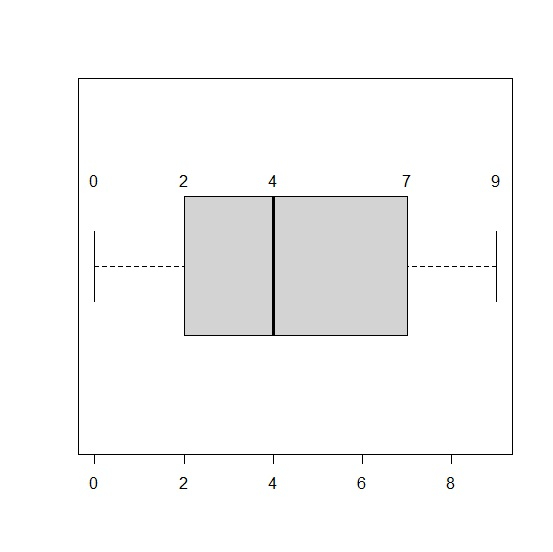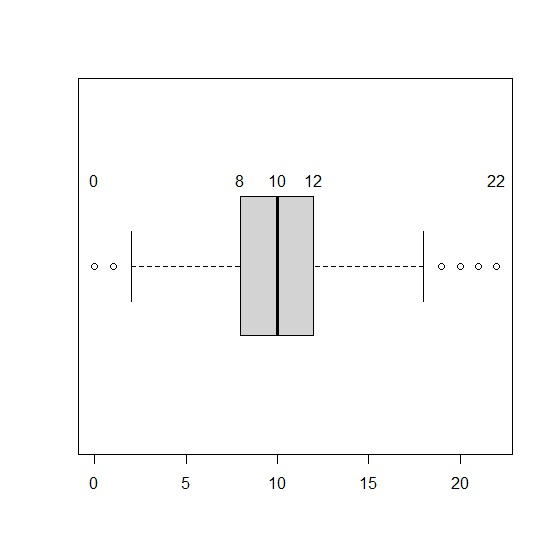# How to show values in boxplot in R?

R ProgrammingServer Side ProgrammingProgramming

#### Artificial Intelligence : The Future Of Programming

15 Lectures 54 mins

#### Beyond Basic Programming - Intermediate Python

Most Popular

36 Lectures 3 hours

#### C Programming from scratch- Master C Programming

Best Seller

60 Lectures 8 hours

The main values in a boxplot are minimum, first quartile, median, third quartile, and the maximum, and this group of values is also called five-number summary. Therefore, if we want to show values in boxplot then we can use text function and provide the five-number summary and labels with fivenum function as shown in the below examples.

## Example1

x<-sample(0:9,500,replace=TRUE)
boxplot(x,horizontal=TRUE) text(x=fivenum(x),labels=fivenum(x),y=1.25)

## Output## Example2

y<-rpois(5000,10)
boxplot(y,horizontal=TRUE)
text(x=fivenum(y),labels=fivenum(y),y=1.25)

## Output